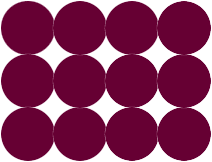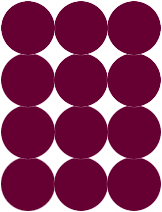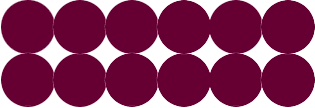Quandaries and Queries My son's fourth grade workbook is asking him to draw four possible arrays for 72 marbles. I don't understand what they mean Danette Hi Danette, The workbook means a rectangular array. I am going to consider 12 rather than 72. Here is a 3 by 4 array of 12 marbles. (Mathematicians always mention the rows first then the columns.)A 4 by 3 array would beor you might have a 2 by 6 arrayArithmetically you are asking to write 12 as the product of two positive integers and then forming an array with these integers. It might even be 1 by 12. In the array all the rows must have the same number of marbles and all the columns must have the same number of marbles. Penny